# Eq2 equations

For each of the following problems, determine the roots of the equation. Given the roots, sketch the graph and explain how your sketch matches the roots given and the form of the equation:
g(x)=36x2-12x+5
h(x)=x2-4x+20
f(x)=4x2-24x+45
p(x)=9x2-36x+40
g(x)=x2-2x+10
g(x)=x2-x-6

g1 =  0
g2 =  0
h1 =  0
h2 =  0

### Step-by-step explanation:

Our quadratic equation calculator calculates it.

Our quadratic equation calculator calculates it.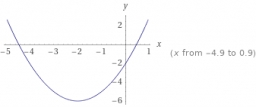Did you find an error or inaccuracy? Feel free to write us. Thank you!Tips to related online calculators

## Related math problems and questions:

• EquationsSolve following system of equations: 6(x+7)+4(y-5)=12 2(x+y)-3(-2x+4y)=-44
• Null points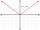Calculate the roots of the equation: 3 |x +4| +3 |x +5| +2 |x +4| = 30
• Profit, revenue, cost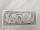Profit, P(x), is the difference between revenue, R(x), and cost, C(x), so P(x) = R(x) - C(x). Which expression represents P(x), if R(x) = 3x3 + 2x - 1 and C(x) = x4 - x2 + 2x + 3?
• Polynomial roots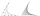Find the other zeroes of the polynomial 2x4+3x3-3x2-6x-2, if two of them are root2 & -root2
• Equation 25Solve following simple equation: 3/4(x+5)=1/2(x+9)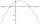It is given a quadratic function y = -4x2+5x+c with unknown coefficient c. Determine the smallest integer c for which the graph of f intersects the x-axis at two different points.
• Domains of functions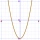F(x)=x2-7x and g(x)=5-x2 Domain of (fg)(x) is. .. . . Domain of (f/g)(x). ..
• Expressions 3If k(x+6)= 4x2 + 20, what is k(10)=?
• Simple equation 6Solve equation with one variable: X/2+X/3+X/4=X+4
• If x=4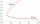If x=4 and y=-3, what is the value of the expression x² + 2xy - 4x + 3y + y²?
• Consider 2Consider the following formula: y = 3 ( x + 5 ) ( x - 2 ) Which of the following formulas is equivalent to this one? A. Y=3x2+9x-30 B. Y=x2+3x-10 C. Y=3x2+3x-10 D. Y=3x2+3x-30
• My fatherMy father has a big farm. 6/8 of it were planted with mango trees, 1/2 of the remainder are guava trees and the rest 10 trees are santol trees. What is the number of all trees?
• ProveProve that k1 and k2 are the equations of two circles. Find the equation of the line that passes through the centers of these circles. k1: x2+y2+2x+4y+1=0 k2: x2+y2-8x+6y+9=0
• Intersections 3Find the intersections of the circles x2 + y2 + 6 x - 10 y + 9 = 0 and x2 + y2 + 18 x + 4 y + 21 = 0
• Trapezium internal anglesA trapezium where AB is parallel to CD, has angle A : angle D = 4 :5, angle B = 3x-15 and angle C = 4x+20. Find angle A, B, C and D.
• Equation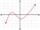Eequation f(x) = 0 has roots x1 = 64, x2 = 100, x3 = 25, x4 = 49. How many roots have equation f(x2) = 0 ?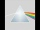Please determine the solvability conditions of the equation, solve the equation and perform the test: x divided by x squared minus 2x plus1 the whole minus x + 3 divided by x squared minus 1 this is equal to 0: x/(x2-2x+1) - (x+3)/( x2-1) = 0